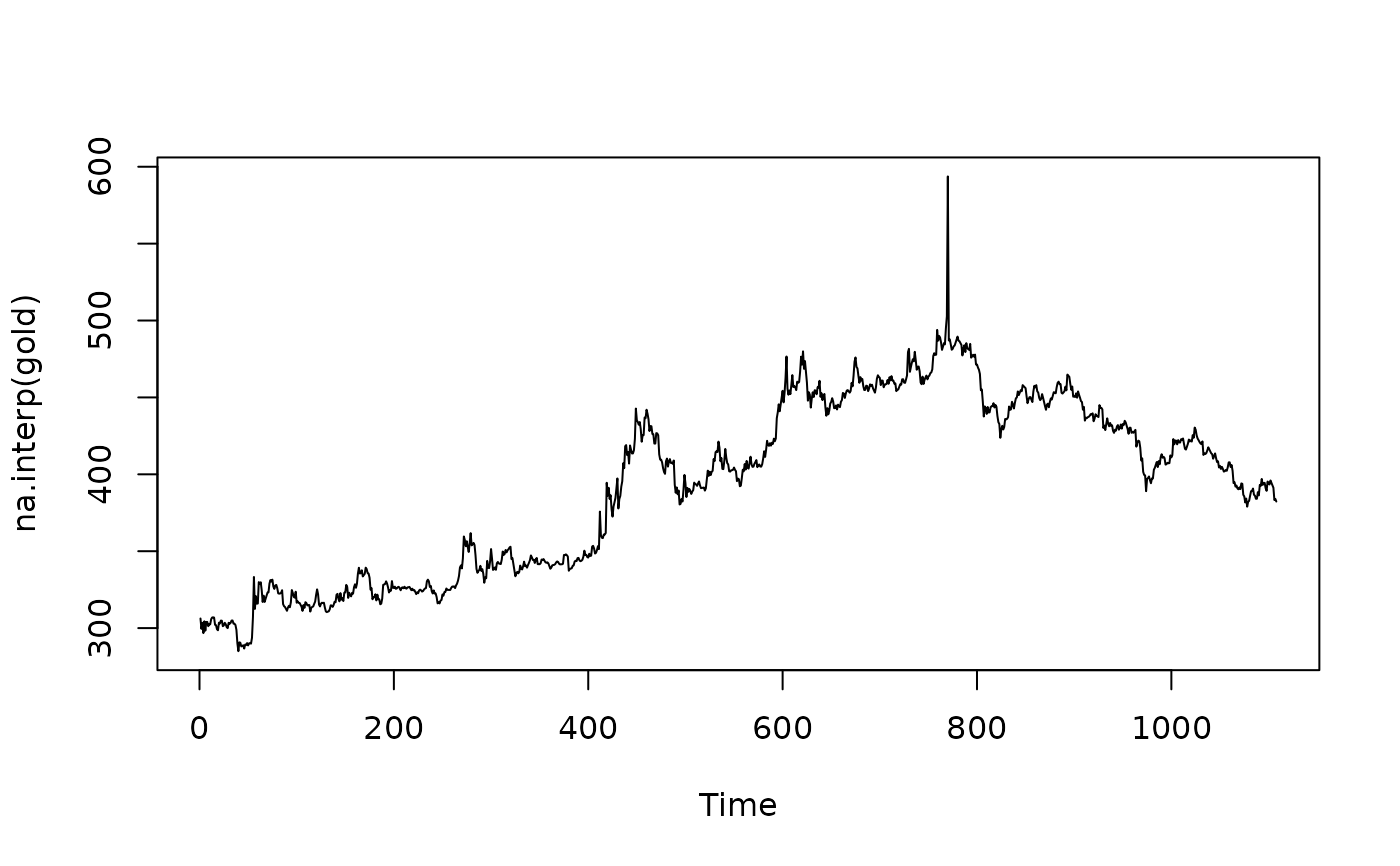By default, uses linear interpolation for non-seasonal series. For seasonal series, a robust STL decomposition is first computed. Then a linear interpolation is applied to the seasonally adjusted data, and the seasonal component is added back.

## Usage

na.interp(
x,
lambda = NULL,
linear = (frequency(x) <= 1 | sum(!is.na(x)) <= 2 * frequency(x))
)

## Arguments

x

time series

lambda

Box-Cox transformation parameter. If lambda="auto", then a transformation is automatically selected using BoxCox.lambda. The transformation is ignored if NULL. Otherwise, data transformed before model is estimated.

linear

Should a linear interpolation be used.

Time series

## Details

A more general and flexible approach is available using na.approx in the zoo package.

tsoutliers

Rob J Hyndman

## Examples


data(gold)
plot(na.interp(gold))